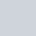# Functions & Predefined FunctionsWritten by

Function is very important , because it reduces the program length . Function is a complete bunch of instructions . In javascript some predefined function are also available for your help, in this post i explain you how to declare function in javascript and also some predefined functions .## Declaration Function

In javascript functions are declared by using function keyword

Basic Syntax

function name(parameter1,…)
{
}
• Then type your function name()
Function is help to programmer to reuse the code and function concept is same as class ,because we create class for reuse the code and in same way we create the function for reuse the code .But the Measure Difference b/w these are calling, class we need to create object and in function is not required

Example

```function add2(num)
{
return num+2;
}
•  Above i create add2 function ,which return +2 in given number.
• And then i calling add2 function and in function i pass of number “4” then i use console.log for see output and  the function output is “6”

This type we declare function and we the call the function..

## Recursive Function

we know that basically in dictionary recursive means again and again ; In same way in recursive functions are those function , which has no loop, but it call function in function body . Let see how

Example

```function fact(n)
{

// The number is less than that means number is not postive
if (n< 0)
{
return "Enter number b/w 0-10 ";
}

else if ((n == 0) || (n == 1))
{ // If the number is 0 or 1, its factorial is 1.
return 1;
}
else
{ // calling main function in same function
return (n * fact(n - 1));
}
}

num = prompt("Enter a number:");
console.log(fact(num));```
• Here i create function Fact() using idea of recursive function .
• And the function asked number using dialog box, because i use prompt method and after enter the number the function check the number in value and then send you your function factorial .

# Predefined Function

Javascript has some predefined function ; here i explain some functions of javascript for see more function you click at this link all function . These functions are following

• isNan()
• isFinite()
• Number and String
• substring()

isNan() : The isNaN() function returns true if the value is not a number, false if the value is a number.

Example

```    var a = isNaN(5);
var b = isNaN("");
console.log(a);//output false
console.log(b);//output true

```

isFinite(): The isFinite() function returns false if the value is not a finite number, we can say the this opposite of isNaN() function

Example

```var a = isFinite(95);
var b = isFinite("howdy") ;
console.log(a);//output true
console.log(b);//output false

```

Number(): The Number()function change the string value into number

Example

```var name = "A",number;
number = Number(name);//output NaN```
• here i try to convert string “A” into a number and the Number function send me NaN in output ,because “A” is not a number .
• If you replace “A” by “1” then output become a number then the value of number  var is 1

String(): The String()function change the number value into string

Example

```var number=NaN,str;
str = String(number);//output is "NaN"

```

substring(): The function use for chopping the string mean it chop the string like you chop vegetables . function substring() want two values i and j , where i for start chopping and j for end chopping .

Example

```var str = "hifriends";
var chop = str.substring(0, 4);
console.log(chop);//output "hifr"```

in:

Tagged:

Previous article

Operators & Control structure

Next article

Loops In Javascript

## Join the discussion

This site uses Akismet to reduce spam. Learn how your comment data is processed.